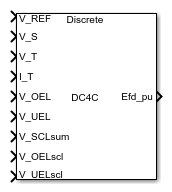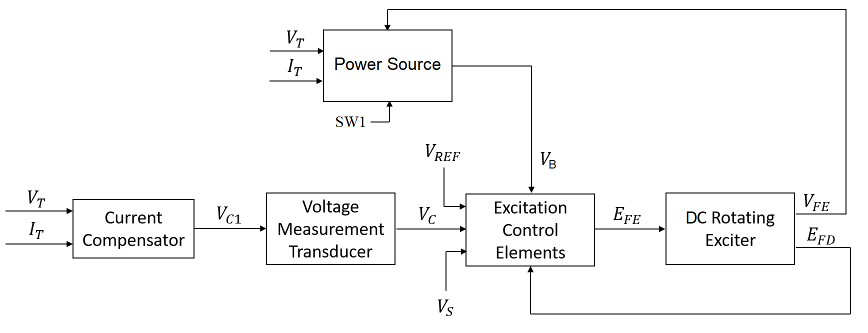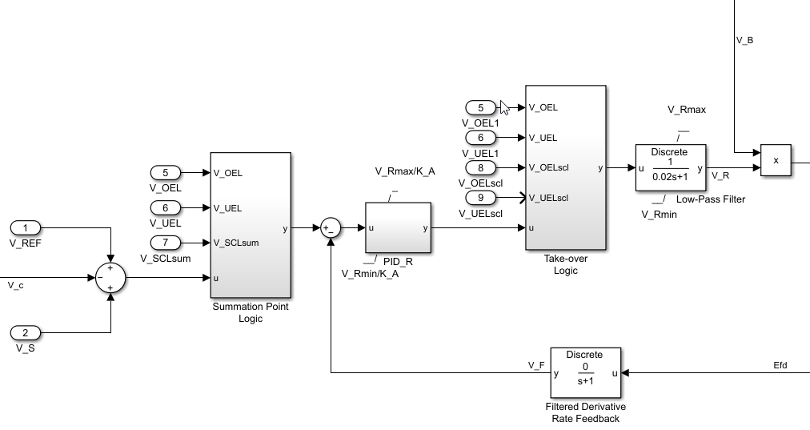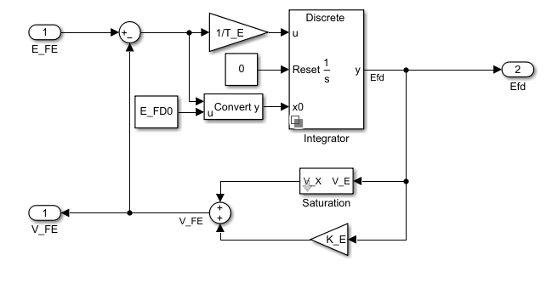# SM DC4C

Discrete-time or continuous-time synchronous machine DC4C excitation system including an automatic voltage regulator and exciter

Since R2021b

•Libraries:
Simscape / Electrical / Control / SM Control

## Description

The SM DC4C block models a synchronous machine type DC4C excitation system that conforms to IEEE 421.5-2016. Use this block to model the control and regulation of the field voltage of a synchronous machine that operates as a generator using a DC commutator rotating exciter.

You can switch between continuous and discrete implementations of the block by using the Sample time (-1 for inherited) parameter. To configure the block for continuous time, set the Sample time (-1 for inherited) property to `0`. To configure the block for discrete time, set the Sample time (-1 for inherited) property to a positive, nonzero value, or to `-1` to inherit the sample time from an upstream block.

The SM DC4C block is made up of five major components:

• The current compensator modifies the measured terminal voltage as a function of terminal current.

• The voltage measurement transducer simulates the dynamics of a terminal voltage transducer by using a low-pass filter.

• The excitation control elements component compares the voltage transducer output with a terminal voltage reference to produce a voltage error. This voltage error is then passed through a voltage regulator to produce the exciter field voltage.

• The DC rotating exciter models the DC commutator rotating exciter which produces and applies a field voltage to the controlled synchronous machine. The block also feeds the field voltage back to the excitation system.

• The power source models the dependency of the power source for the controlled rectifier from the terminal voltage.

This diagram shows the overall structure of the DC4C excitation system model:In the diagram:

• VT and IT are the measured terminal voltage and current of the synchronous machine.

• VC1 is the current-compensated terminal voltage.

• VC is the filtered, current-compensated terminal voltage.

• VREF is the reference terminal voltage.

• VS is the power system stabilizer voltage.

• SW1 is the user-selected power source switch for the controlled rectifier.

• VB is the exciter field voltage.

• EFE and VFE are the exciter field voltage and current, respectively.

• EFD is the field voltage.

### Current Compensator and Voltage Measurement Transducer

The current compensator is modeled as:

`${V}_{C1}={V}_{T}+{I}_{T}\sqrt{{R}_{C}^{2}+{X}_{C}^{2}},$`

where:

• RC is the load compensation resistance.

• XC is the load compensation reactance.

The voltage measurement transducer is implemented as a Low-Pass Filter block with the time constant TR. To see the exact discrete and continuous implementations, see Low-Pass Filter.

### Excitation Control Elements

This diagram illustrates the overall structure of the excitation control elements:In the diagram:

• The Summation Point Logic subsystem models the summation point input location for the overexcitation limiter (OEL), underexcitation limiter (UEL), and stator current limiter (SCL) voltages. For more information about using limiters with this block, see Field Current Limiters.

• The Low-Pass Filter block models the major dynamics of the voltage regulator. Here, KA is the regulator gain and TA is the major time constant of the regulator. The minimum and maximum anti-windup saturation limits for the block are VRmin and VRmax, respectively.

• The PID_R subsystem models a PID controller that functions as a control structure for the automatic voltage regulator. The minimum and maximum anti-windup saturation limits for the block are VRmax / KA and VRmin / KA, respectively.

• The Take-over Logic subsystem models the take-over point input location for the OEL, UEL, and SCL voltages. For more information about using limiters with this block, see Field Current Limiters.

• The Filtered Derivative block models the rate feedback path for stabilization of the excitation system. Here, KF and TF are the gain and time constant of this system, respectively. To see the exact discrete and continuous implementations, see Filtered Derivative.

### Field Current Limiters

You can use various field current limiters to modify the output of the voltage regulator under unsafe operating conditions:

• Use an overexcitation limiter to prevent overheating of the field winding due to excessive field current demand.

• Use an underexcitation limiter to boost field excitation when it is too low, which can risk desynchronization.

• Use a stator current limiter to prevent overheating of the stator windings due to excessive current.

Attach the output of any of these limiters at one of these points:

• The summation point as part of the automatic voltage regulator (AVR) feedback loop

• The take-over point to override the usual behavior of the AVR

If you are using the stator current limiter at the summation point, use the single input V_SCLsum. If you are using the stator current limiter at the take-over point, use both an overexcitation input V_OELscl and an underexcitation input V_UELscl.

### DC Rotating Exciter

This diagram illustrates the overall structure of the DC commutator rotating exciter:In the diagram:

• The exciter field current VFE is the sum of two signals:

• The nonlinear function Vx models the saturation of the exciter output voltage.

• The proportional term KE models the linear relationship between exciter output voltage and the exciter field current.

• The Integrator subsystem integrates the difference between EFE and VFE to generate the output field voltage Efd. TE is the time constant for this process.

### Power Source

You can change the power source representations for the controlled rectifier with the Logical switch 1 parameter. The power source for the controlled rectifier can be either derived from the terminal voltage (```Position A: power source derived from terminal voltage```) or it can be independent of the terminal voltage (```Position B: power source independent from the terminal conditions```).## Ports

### Input

expand all

Voltage regulator reference set point, in per-unit representation, specified as a scalar.

Data Types: `single` | `double`

Input from the power system stabilizer, in per-unit representation, specified as a scalar.

Data Types: `single` | `double`

Terminal voltage magnitude, in per-unit representation, specified as a scalar.

Data Types: `single` | `double`

Terminal current magnitude, in per-unit representation, specified as a scalar.

Data Types: `single` | `double`

Input from the overexcitation limiter, in per-unit representation, specified as a scalar.

• To ignore the input from the overexcitation limiter, set Alternate OEL input locations (V_OEL) to `Unused`.

• To use the input from the overexcitation limiter at the summation point, set Alternate OEL input locations (V_OEL) to ```Summation point```.

• To use the input from the overexcitation limiter at the take-over point, set Alternate OEL input locations (V_OEL) to `Take-over`.

Data Types: `single` | `double`

Input from the underexcitation limiter, in per-unit representation, specified as a scalar.

• To ignore the input from the underexcitation limiter, set Alternate UEL input locations (V_UEL) to `Unused`.

• To use the input from the underexcitation limiter at the summation point, set Alternate UEL input locations (V_UEL) to ```Summation point```.

• To use the input from the underexcitation limiter at the take-over point, set Alternate UEL input locations (V_UEL) to `Take-over`.

Data Types: `single` | `double`

Input from the stator current limiter when using the summation point, in per-unit representation, specified as a scalar.

• To ignore the input from the stator current limiter, set Alternate SCL input locations (V_SCL) to `Unused`.

• To use the input from the stator current limiter at the summation point, set Alternate SCL input locations (V_SCL) to ```Summation point```.

Data Types: `single` | `double`

Input from the stator current limiter to prevent field overexcitation when using the take-over point, in per-unit representation, specified as a scalar.

• To ignore the input from the stator current limiter, set Alternate SCL input locations (V_SCL) to `Unused`.

• To use the input from the stator current limiter at the take-over point, set Alternate SCL input locations (V_SCL) to `Take-over`.

Data Types: `single` | `double`

Input from the stator current limiter to prevent field underexcitation when using the take-over point, in per-unit representation, specified as a scalar.

• To ignore the input from the stator current limiter, set Alternate SCL input locations (V_SCL) to `Unused`.

• To use the input from the stator current limiter at the take-over point, set Alternate SCL input locations (V_SCL) to `Take-over`.

Data Types: `single` | `double`

### Output

expand all

Per-unit field voltage to apply to the field circuit of the synchronous machine, returned as a scalar.

Data Types: `single` | `double`

## Parameters

expand all

### General

Initial per-unit voltage to apply to the field circuit of the synchronous machine.

Initial per-unit terminal voltage.

Initial per-unit terminal current.

Time between consecutive block executions. During execution, the block produces outputs and, if appropriate, updates its internal state. For more information, see What Is Sample Time? and Specify Sample Time.

For inherited discrete-time operation, set this parameter to `-1`. For discrete-time operation, set this parameter to a positive integer. For continuous-time operation, set this parameter to `0`.

If this block is in a masked subsystem or a variant subsystem that supports switching between continuous operation and discrete operation, promote this parameter to ensure correct switching between the continuous and discrete implementations of the block. For more information, see Promote Block Parameters on a Mask.

### Pre-Control

Resistance used in the current compensation system, in per-unit. Set this parameter and Reactance component of load compensation, X_C (pu) to `0` to disable current compensation.

Reactance used in the current compensation system, in per-unit. Set this parameter and Resistive component of load compensation, R_C (pu) to `0` to disable current compensation.

Equivalent time constant for the voltage transducer filtering, in seconds.

### Control

Per-unit proportional gain of the regulator.

Per-unit integral gain of the regulator.

Derivative gain of the regulator.

Equivalent filter time constant for the derivative channel of the PID controller, in seconds.

Gain associated with the voltage regulator.

Major time constant of the voltage regulator, in seconds.

Rate feedback block gain for the stabilization of the excitation system.

Rate feedback block time constant for the stabilization of the excitation system, in seconds.

Maximum per-unit output voltage of the controller.

Minimum per-unit output voltage of the controller.

Location of the overexcitation limiter input.

• To ignore the input from the overexcitation limiter, set Alternate OEL input locations (V_OEL) to `Unused`.

• To use the input from the overexcitation limiter at the summation point, set Alternate OEL input locations (V_OEL) to ```Summation point```.

• To use the input from the overexcitation limiter at the take-over point, set Alternate OEL input locations (V_OEL) to `Take-over`.

Location of the underexcitation limiter input.

• To ignore the input from the underexcitation limiter, set Alternate UEL input locations (V_UEL) to `Unused`.

• To use the input from the underexcitation limiter at the summation point, set Alternate UEL input locations (V_UEL) to ```Summation point```.

• To use the input from the underexcitation limiter at the take-over point, set Alternate UEL input locations (V_UEL) to `Take-over`.

Location of the stator current limiter input. To specify the stator current limiter input:

• If you select `Summation point`, use the V_SCLsum input port.

• If you select `Take-over`, use the V_OELscl and V_UELscl input ports.

### Exciter

Proportional constant for the exciter field.

Time constant for the exciter field, in seconds.

Lower limit for the field voltage.

Exciter output voltage for the first saturation factor.

First exciter saturation factor.

Exciter output voltage for the second saturation factor.

Second exciter saturation factor.

Per-unit potential circuit gain coefficient.

Potential circuit phase angle, in degrees.

Per-unit potential circuit current gain coefficient.

Per-unit reactance associated with the potential source.

Per-unit loading factor of the rectifier that is proportional to the commutating reactance.

Position of logical switch 1.

Maximum per-unit available field voltage for the exciter.

 IEEE Recommended Practice for Excitation System Models for Power System Stability Studies. IEEE Std 421.5-2016. Piscataway, NJ: IEEE-SA, 2016.